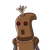# (a) Every natural number is a rational number but every rational numberneeds not be a natural number. (b) Zero is a ra

(a) Every natural number is a rational number but every rational number

needs not be a natural number. (b) Zero is a rational number. (c) Every rational number is a whole number. (d) Two rational numbers with difference numerators can’t be equal. (e) Every fraction is a rational number. (f) Sum of two rational numbers is always a rational number. (g) The rational number -3/5 lies to the right of zero on the number line. (h) Every natural number is a rational number but every rational number need

not be a natural number. (I) 2/4 is equivalent to 4/8

(j) The rational numbers -11/-12 and -7/8 are on the opposite sides of zero on

the number line​

### 1 thought on “(a) Every natural number is a rational number but every rational number<br /><br />needs not be a natural number. (b) Zero is a ra”

1.